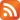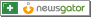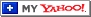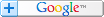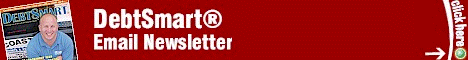Web DebtSmart.comTuesday, May 18, 2021
 How Do You Get that Loan Formula? by Scott Bilker
 Scott Bilker is the author of the best-selling books, Talk Your Way Out of Credit Card Debt, Credit Card and Debt Management, and How to be more Credit Card and Debt Smart. He's also the founder of DebtSmart.com. More about Scott Bilker and DebtSmart can be found in the online media kit.Scott,

I read with interest your article, "Amortized Loans." At the end of the article you indicate the explanation of how to derive the formulae as being covered in another article.

Can you please tell me where I can find this?

Thank you very much,
Luke

Luke,

Here is the derivation of the loan formula from scratch:

F --> Future Value
P --> Principal
A --> Annuity
n --> period number
i --> interest

#1) F0=P
Simply states that the future value at time 0 is equal to the principal.

#2) F1=P+Pi-A=P(1+i)-A
The future value, after one period, is equal to the principal (P) with the addition of the interest in dollars (Pi) and then you subtract the periodic payment (A). The second part of the equation factors P from P+Pi. This step is repeated for all periods.

#3) F2=F1(1+i)-A=P((1+i)^2)-A(1+i)-A
The future value, after the second period is equal to the new balance (F1) with the addition of the interest in dollars (F1i) and then you subtract the periodic payment (A). The second part of the equation substitutes P(1+i)-A for F1 (from equation #2).

#4) F3=F2(1+i)-A=P((1+i)^3)-A((1+i)^2)-A(1+i)-A
Applying the same procedure in steps #2 and #3 we get the future value at period #3.

#5) F4=F3(1+i)-A=P((1+i)^4)-A((1+i)^3)-A((1+i)^2)-A(1+i)-A
Applying the same procedure in steps #2 and #3 we get the future value at period #4.

#6) Fn=P((1+i)^n)-A[((1+i)^(n-1))+((1+i)^(n-2))+((1+i)^(n-3))+....+1]
This is the future value at period n, or any period, based on the general equation being expanded. Additionally, A is factored from all terms.The first part of #6, P((1+i)^n), is easy to work with because it is a closed form, however, we need to find the closed form for the remaining part of that formula, therefore, for the general case of [((1+i)^(n-1))+((1+i)^(n-2))+((1+i)^(n-3))+....+1]:

#7) y=1+a^1+a^2+a^3+a^4+a^5+a^6+....a^k
This formula is very similar to the case we are trying to solve because it's the addition of a to all powers from 0 to k.

#8) y-1=a^1+a^2+a^3+a^4+a^5+a^6+....a^k
Subtracting 1 from both sides.

#9) (y-1)/a=1+a^1+a^2+a^3+a^4+a^5+....a^(k-1)
Dividing all terms by a.

#10) (y-1)/a+a^k=1+a^1+a^3+a^3+a^4+a^4+a^5+....a^k
Adding a^k to both sides because...

#11) 1+a^1+a^2+a^3+a^4+a^5+....a^k=y
we get the very same equation we started with (#7).

Therefore,

#12) (y-1)/a+a^k=y
Substituting #7 into #10.

#13) y-1+a^(k+1)=ya
Multiplying all terms by a.

#14) y-ya=1-a^(k+1)
Putting like terms on opposite sides of the equation.

#15) y(1-a)=1-a^(k+1)
Factoring y out from left side.

#16) y=(1-a^(k+1))/(1-a)
Solving for y.

Going back to the general case for the loan formula in #6:
#17) [((1+i)^(n-1))+((1+i)^(n-2))+((1+i)^(n-3))+....+1]

#18) y=[((1+i)^(n-1))+((1+i)^(n-2))+((1+i)^(n-3))+....+1]
Set y equal to #17.

#19) y=(1-a^(k+1))/(1-a)
This is the solution to #18 based on #16 where k=n-1 (the highest power in #18) and a=1+i.

#20) y=(1-((1+i)^n))/(-i)
Substitute k=n-l and a=1+i in #19.

#21) y=(((1+i)^n)-1)/I
Multiplying numerator and denominator by -1.

Back to #6:
#22) Fn=P((1+i)^n)-A[((1+i)^(n-1))+((1+i)^(n-2))+((1+i)^(n-3))+....+1]

#23) Fn=P((1+i)^n)-A[y]
Substituting y from equation defined in #18.

#24) Fn=P((1+i)^n)-A[(1-((1+i)^n))/i]
Substituting again for y in equation #21.

Note: Fn=0, the last future value of the loan, when the loan is repaid, therefore,

#25) 0=P((1+i)^n)-A[(((1+i)^n)-1)/i] (at period n)
Substituting 0 for Fn.

#26) A[(((1+i)^n)-1)/i]=P((1+i)^n)
Separating terms with A and P on both sides of the equation.

#27) A=[P((1+i)^n)][i/(((1+i)^n)-1)]
Solving for A.

#27) A=Pi/(1-((1+i)^(-n)))
Simplifying terms by multiplying numerator and denominator by ((1+i)^(-n)).

We have it! Equation #27 is the formula used to calculate the periodic payment (A) for a loan of principal (P) at the periodic rate (i) for n periods.

Here's a quick example:
What is the monthly payment for a \$10,000 loan with an APR of 24% for 5 years?

Solution:
P=10000
i=0.24/12=0.02 (period is monthly so 2% is the monthly rate)
n=5(12)=60 months or 60 periods

Formula is:
A=Pi/(1-((1+i)^(-n)))

A=10000(0.02)/(1-((1+0.02)^(-60)))

A=200/(1-0.30478)=200/0.69522

A=287.68

Therefore, the monthly payment is \$287.68.

I hope you enjoyed this derivation!

Regards,
Scott

--End--

 Subscribe FREE and start finding new ways to save money and pay off your debt. "The DebtSmart Email Newsletter is packed with cutting-edge strategies for solving credit problems. I highly recommend it."--Gerri Detweiler, radio host and author of The Ultimate Credit HandbookDEBTSMART MEDIA MENTIONSNBC 10 News: Money King Secrets CN8:Art Fennell Reports CNN: CNN Newsroom CNN: American Morning ABC: Action News CNN/fn: Your Money

 Subscribe to the DebtSmart® RSS Feed